#### Cusp and Generalized Hopf bifurcations

Here the bifurcations concern singularities with co-dimension greater than 1. These are only unfolded by local families that depend on more than 1 parameter.

##### Cusp bifurcation

Given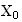a smooth vector field with a cusp singularity at the origin. Then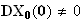but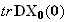=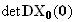=andhas normal form (with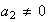and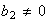).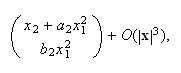The local family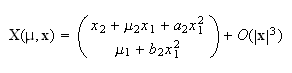is a versal unfolding of the cusp singularity above. (Note that the cusp singularity requires at least two parameters to completely unfold it). Considering this local family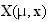, under some conditions (not shown here) in particular about parameters, it is possible to generate three bifurcation types: (1) Saddle node, (2) Hopf bifurcation, (3) Saddle connection.

##### Generalized Hopf bifurcations

Generalized Hopf bifurcations. (Takens 1973b, Guckenheimer & Holmes 1983, Arrowsmith & Place 1990). Here, we describe the generalized Hopf singularity of type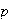. The vector field in the previous co-dimension 1 Hopf bifurcation was obtained by posing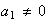in the normal form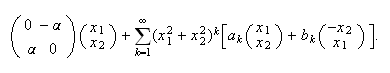If a degeneracy is introduced by posing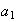=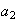=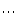=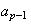=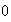with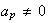, then the system above is said to have a generalized Hopf singularity of typeat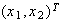=. (Note that a co-dimension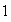Hopf singularity is said to be of type). The framework of the generalized Hopf singularity highlights the relationship between the degeneracy of a singularity and the number of parameters contained in its versal unfolding.

If a Hopf singularity of typeoccurs, it is possible to reduce the system above to a simpler system. Given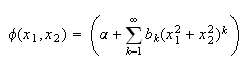that can also be written: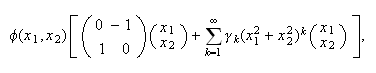with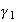=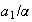. Because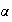>, we have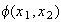>for allenough close to the origin. Thus there exists a neighborhood of=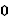on which the system is topologically equivalent to the (simpler) vector field: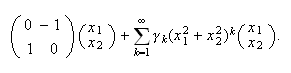While preserving the topological equivalence, we can replaceby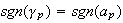in the vector field above. Two cases are then possible according to a singularity of the type: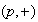or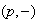. A versal unfolding for the vector field above can be obtained through the following Takens theorem (1973b):

Theorem

The versal unfolding of a generalized Hopf singularity of typegiven in the simpler vector field above is written (where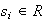,=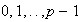):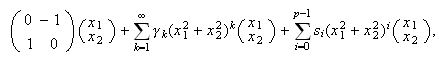According to the sign of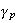, the unfoldings of in this theorem gives two casesand. Here, we only describe the first case, and the latter case can be related to the other by a time reversal (so the stabilities of fixed points and limit cycles are inverted). The formula in this theorem, through the polar form (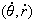with here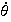=), gives forthe bifurcational behavior by using the equivalent equation: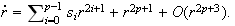The behavior is independent of the terms of order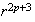(as described for the co-dimension 1 Hopf bifurcation), so it suffices to consider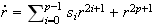. This equation in particular provides limit cycles. (1) For=, by posing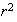=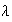, we obtain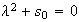, then a limit cycle occurs whose radius is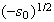only when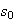<. (2) For=, by posing=, we obtain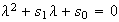and non-trivial zeros result from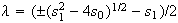. Then we obtain:If>and>(or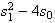<): no limit cycle but a repelling spiral.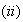If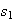<and>: two limit cycles.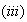If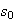<: one limit cycle. (see Fig.below).This figure shows the bifurcation diagram for the type-(2,+) Hopf bifurcation. It shows that a supercritical (subcritical) Hopf bifurcation occurs whenincreases through zero with<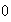(subcritical with>), and a double limit cycle bifurcation occurs on the semi-parabola. Ifdecreases through the semi-parabola, a non-hyperbolic limit cycle occurs and then divides into two hyperbolic cycles.